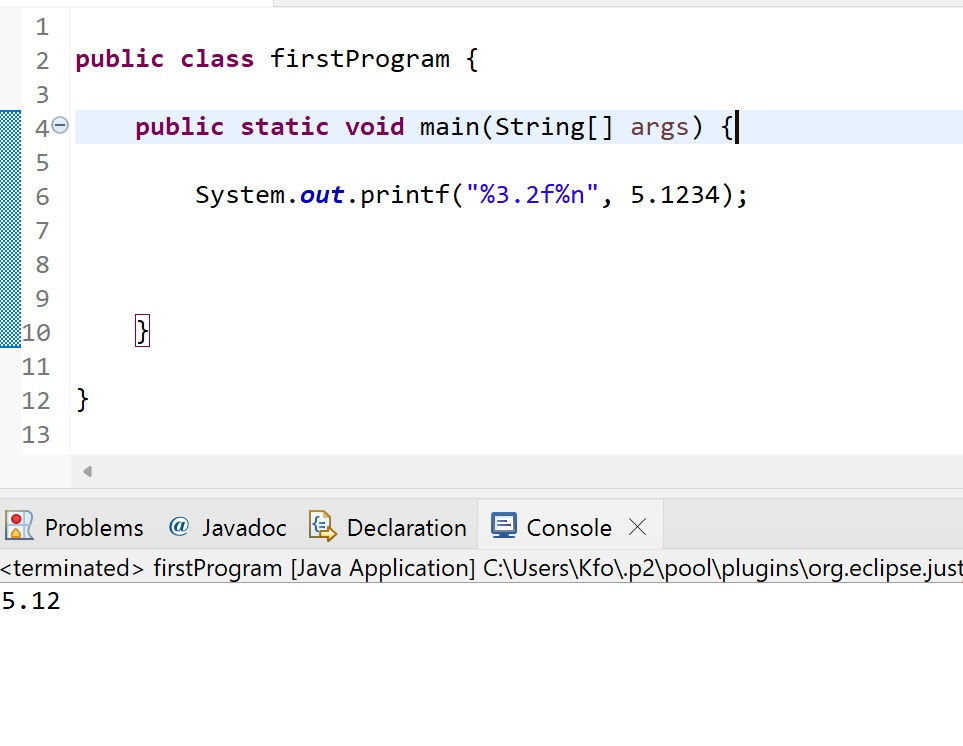•A.razaq

# T6- printf method

printf is used to display formatted data using format specifiers

• %d decimal (integer) number (base 10)

• %f floating-point number

• %s String

```System.out.printf(string);
System.out.printf(format, arguments);   ```

The string parameter is simple as the printIn() method.

The second line would be used to format the output where the argument would be the string that we want to format and the format parameter determines the formatting that will occur.

so in the next example we will use the s specifier for formatting Strings

```System.out.printf("%s\n", "Example one");
System.out.printf("%S\n", "example one");```

we used the s specifier in a lower case and new line character \n

the second one we used uppercase String S specifier

the output will be like this

```Example one
EXAMPLE ONE```now lets use another format specifier and in this case lets go with the integer

```int x = 10;

System.out.printf("integer example : %d %d %n", x, -x); ```

%d %d because we have 2 integers and %n for a new line character just like \n

the output will benow for floating numbers to format a float number, we have to use the %f specifier.

`System.out.printf("%f%n", 5.1234);`

the result will beas we can see the number of decimal places by default is always 6 decimal places

and we can change that by using a precision formatting

`System.out.printf("%3.2f%n", 5.1234); `

the minimum width of our floating number will be 3,

and the length of the decimal will be 2 so the output will be